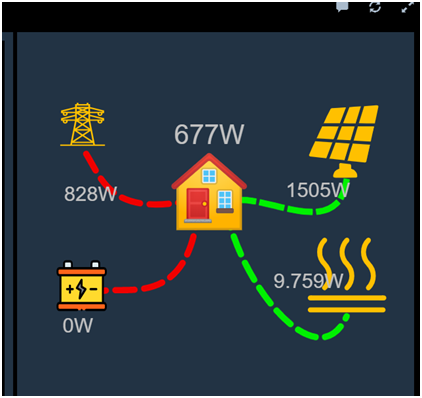# Way to customize solar energy pannel

I’v found a way to customize my solar energy pannel in Openhab3,I’d like to share it with you.

I’m using this folked version:GitHub - lewei50/habpanel-powerflow-widget: SVG based widget to display power flow

Step1:get all data from your device,I am using IamMeter Three Phase Wi-Fi Energy Meter

it provides data from http GET method.

we can get data using “HTTP Binding” or “IamMeter Binding” in Openhab,just follow instructions from iammeter website

I am using HTTP Binding as example,using these codes below to fetch data to items:

``````UID: http:url:cd4259d574

label: solarurl

thingTypeUID: http:url

configuration:

authMode: BASIC

ignoreSSLErrors: false

baseURL: https://www.iammeter.com/api/v1/site/meterdata/YOUR_SN?token=your_token_on_web_site

delay: 0

stateMethod: GET

refresh: 30

commandMethod: GET

contentType: application/json

timeout: 3000

bufferSize: 2048

channels:

\- id: solar_voltage_a

channelTypeUID: http:number

label: Voltage

description: null

configuration:

stateTransformation: JSONPATH:\$.data.values

unit: V

\- id: solar_current_a

channelTypeUID: http:number

label: Current

description: null

configuration:

stateTransformation: JSONPATH:\$.data.values

unit: A

\- id: solar_power_a

channelTypeUID: http:number

label: Power

description: null

configuration:

stateTransformation: JSONPATH:\$.data.values

unit: W

\- id: solar_importenergy_a

channelTypeUID: http:number

label: Importenergy

description: null

configuration:

stateTransformation: JSONPATH:\$.data.values

unit: kWh

\- id: solar_exportgrid_a

channelTypeUID: http:number

label: Exportgrid

description: null

configuration:

stateTransformation: JSONPATH:\$.data.values

unit: kWh

\- id: solar_voltage_b

channelTypeUID: http:number

label: Voltage

description: null

configuration:

stateTransformation: JSONPATH:\$.data.values

unit: V

\- id: solar_current_b

channelTypeUID: http:number

label: Current

description: null

configuration:

stateTransformation: JSONPATH:\$.data.values

unit: A

\- id: solar_power_b

channelTypeUID: http:number

label: Power

description: null

configuration:

stateTransformation: JSONPATH:\$.data.values

unit: W

\- id: solar_importenergy_b

channelTypeUID: http:number

label: Importenergy

description: null

configuration:

stateTransformation: JSONPATH:\$.data.values

unit: kWh

\- id: solar_exportgrid_b

channelTypeUID: http:number

label: Exportgrid

description: null

configuration:

stateTransformation: JSONPATH:\$.data.values

unit: kWh

\- id: solar_voltage_c

channelTypeUID: http:number

label: Voltage

description: null

configuration:

stateTransformation: JSONPATH:\$.data.values

unit: V

\- id: solar_current_c

channelTypeUID: http:number

label: Current

description: null

configuration:

stateTransformation: JSONPATH:\$.data.values

unit: A

\- id: solar_power_c

channelTypeUID: http:number

label: Power

description: null

configuration:

stateTransformation: JSONPATH:\$.data.values

unit: W

\- id: solar_importenergy_c

channelTypeUID: http:number

label: Importenergy

description: null

configuration:

stateTransformation: JSONPATH:\$.data.values

unit: kWh

\- id: solar_exportgrid_c

channelTypeUID: http:number

label: Exportgrid

description: null

configuration:

stateTransformation: JSONPATH:\$.data.values

unit: kWh
``````

Step2:Calculate data from raw data.

all data above were raw data,and can’t be displayed in Pannel directly.we use RULES in Openhab system to calculate them.

``````rule "Update Energy Usage"

when

Time cron "0 0 0 * * ? *"

then

end

rule "Update Solar Energy Today"

when

​      //Item solar_power_a changed

​      Time cron "0 * * * * ?" //for debug

then

​      //var Number feedin_power_tmp           = (solar_power_b.state as Number) * (-1|W)

​      var load_power_tmp           = ( (solar_power_a.state as Number) + (solar_power_b.state as Number) )* (1|W)

​      inverter_power.postUpdate(solar_power_a.state)

​      feedin_power.postUpdate( (solar_power_b.state as Number) * (-1|W) )

​      grid_consumption_energy.postUpdate(solar_importenergy_b.state)

​      exported_energy.postUpdate(solar_exportgrid_b.state)

​      yield_energy.postUpdate(solar_importenergy_a.state)

​      var selfuse_energy_tmp  = ((yield_energy.state as Number).floatValue - (exported_energy.state as Number).floatValue)*(1|kWh)

​      var load_energy_tmp          = ((grid_consumption_energy.state as Number).floatValue + (yield_energy.state as Number).floatValue - (exported_energy.state as Number).floatValue)*(1|kWh)

​      selfuse_energy.postUpdate( selfuse_energy_tmp )

​      var Number self_consumption_rate_daily_tmp

​      var Number self_consumption_rate_monthly_tmp

​      var grid_consumption_energy_now = ((grid_consumption_energy.state as Number).floatValue)*(1|kWh)

​      var grid_consumption_energy_daily_min = (grid_consumption_energy.minimumSince(now.with(LocalTime.of(0,0,0,0)),"rrd4j").state as Number)*(1|kWh)

​      grid_consumption_energy_daily.postUpdate((grid_consumption_energy_now - grid_consumption_energy_daily_min))

​      var exported_energy_now = (exported_energy.state as Number)*(1|kWh)

​      var exported_energy_daily_min = (exported_energy.minimumSince(now.with(LocalTime.of(0,0,0,0)),"rrd4j").state as Number)*(1|kWh)

​      exported_energy_daily.postUpdate((exported_energy_now - exported_energy_daily_min))

​      var yield_energy_now = (yield_energy.state as Number)*(1|kWh)

​      var yield_energy_daily_min = (yield_energy.minimumSince( now.with(LocalTime.of(0,0,0,0)),"rrd4j").state as Number)*(1|kWh)

​      yield_energy_daily.postUpdate((yield_energy_now - yield_energy_daily_min))

​      var selfuse_energy_daily_min = (selfuse_energy.minimumSince( now.with(LocalTime.of(0,0,0,0)),"rrd4j").state as Number)*(1|kWh)

​      selfuse_energy_daily.postUpdate((selfuse_energy_tmp - selfuse_energy_daily_min))

end
``````

Step3:Configuation pannel

Add widget in Page configuation of Openhab,import from file “power-flow.widget.json” that we cloned just now.

copy directory “powerFlow” to your Openhab(openhab\conf\html)

after that,you can add this widget “Power Flow”.

config it with: